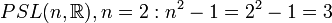# Projective special linear group:PSL(2,R)

View a complete list of particular groups (this is a very huge list!)[SHOW MORE]

## Definition

### Definition as a matrix group$PSL(2,\R)$ is defined as the projective special linear group of degree two over the field of real numbers.

In other words, it is defined as$SL(2,\R)/\{ \pm I \}$.

### Definition as a group of fractional linear transformations

The group$PSL(2,\R)$ can be defined as the group of conformal automorphisms of the upper half-plane. In other words, it is the group of maps of the form:$z \mapsto \frac{az + b}{cz + d}$.

where$a,b,c,d \in \mathbb{C}$ are such that these maps send the upper half-plane to itself.

## Arithmetic functions

Function Value Similar groups Explanation
order of a group cardinality of the continuum Same infinite cardinality as its double cover SL(2,R).
exponent of a group infinite Same as its double cover SL(2,R).
composition length 1 groups with same composition length Follows from being simple -- see projective special linear group is simple.
chief length 1 groups with same chief length Follows from being simple -- see projective special linear group is simple.
dimension of an algebraic group 3 groups with same dimension of an algebraic group As$PSL(n,\_), n = 2: n^2 - 1 = 2^2 - 1 = 3$
dimension of a real Lie group 3 groups with same dimension of a real Lie group As$PSL(n,\R), n = 2: n^2 - 1 = 2^2 - 1 = 3$